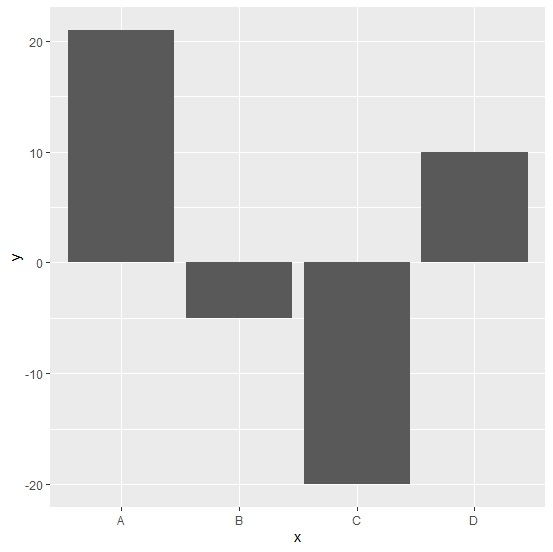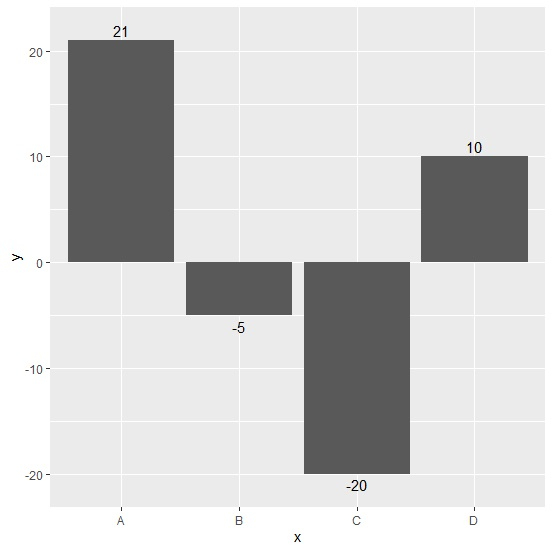# How to display negative labels below bars in barplot using ggplot2 in R?

R ProgrammingServer Side ProgrammingProgramming

#### Artificial Intelligence : The Future Of Programming

15 Lectures 54 mins

#### Beyond Basic Programming - Intermediate Python

Most Popular

36 Lectures 3 hours

#### C Programming from scratch- Master C Programming

Best Seller

60 Lectures 8 hours

Be default, the labels on the plot are represented without sign in a barplot that is created by using ggplot2 but we might want to display the sign of the labels especially in cases where we have some negative values. This can be done with the help of geom_text function of ggplot2 package as shown in the below example.

Consider the below data frame −

## Example

Live Demo

x<-c("A","B","C","D")
y<-c(21,-5,-20,10)
df<-data.frame(x,y)
df

## Output

   x   y
1  A   21
2  B  -5
3  C  -20
4  D   10

Loading ggplot2 package and creating bar chart for data in df −

## Example

library(ggplot2)
ggplot(df,aes(x,y))+geom_bar(stat="identity")

## OutputCreating bar chart for data in df with negative labels displayed with negative sign −

## Example

ggplot(df,aes(x,y))+geom_bar(stat="identity")+geom_text(aes(y=y+sign(y),label=y))

## Output